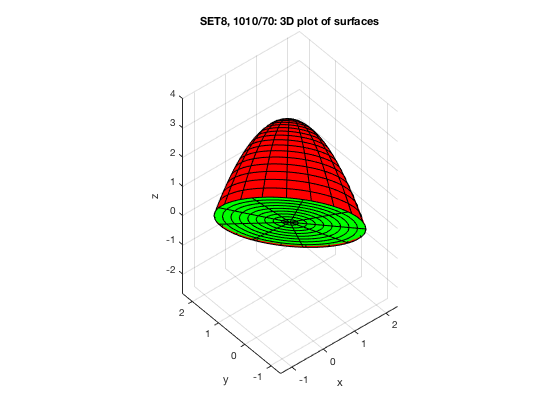# MATH 251: Calculus 3, SET8

## 15: Multiple Integrals

### 15.2: Double Integrals Over General Regions

These problems are done with the CAS. See Hand Solutions for details.

#### 1. [1008/6]

An integral is dispatched.
syms v w
I = int(int(sqrt(1+exp(v)), w, 0, exp(v)), v, 0, 1)
I =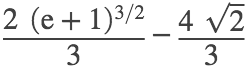I_appx = double(I)
I_appx = 2.8943

#### 2. [1008/14]

The curves intersect at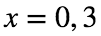. The line is above the parabola.
syms x y
f = x^2, g = 3*x
f =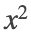g =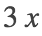figure
fplot(f, [0 3], 'LineWidth', 2); grid on; hold on
fplot(g, [0 3], 'LineWidth', 2)
xlabel('x'); ylabel('y')
axis([0 3 0 9]); xticks(0:3); yticks(0:3:9)
title('SET8, 1008/14: boundary curves')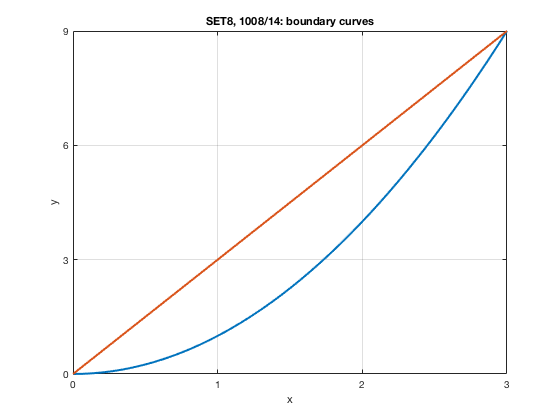%
I1 = int(int(x*y, y, x^2, 3*x), x, 0, 3)
I1 =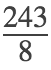I1_appx = double(I1)
I1_appx = 30.3750
I2 = int(int(x*y, x, y/3, sqrt(y)), y, 0, 9)
I2 =I2_appx = 30.3750

#### 3. [1008/20]

An integral over a quarter-circular disk is computed.
syms x y
figure
t = linspace(0,pi/2); xt = cos(t); yt = sin(t);
X = [0 xt 0]; Y = [0 yt 0];
fill(X, Y, 'y'); grid on ; hold on
plot(X, Y, 'LineWidth', 2)
plot([-0.5 1.5], [0 0], 'k')
plot([0 0], [-0.5 1.5], 'k')
xlabel('x'); ylabel('y')
xticks(0:1); yticks(0:1)
axis equal; axis([-0.5 1.5 -0.5 1.5])
title('SET8, 1008/20')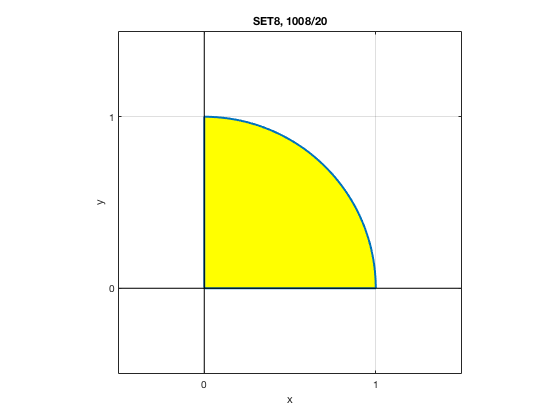%
I = int(int(x*y, y, 0, sqrt(1-x^2)), x, 0, 1)
I =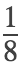I_appx = double(I)
I_appx = 0.1250

#### 4. [1008/28]

The volume of a solid is computed.
syms x y
figure
xs = [0 1 0 0]; ys = [0 1 2 0];
fill(xs, ys, 'y'); grid on; hold on
plot(xs, ys, 'b', 'LineWidth', 2)
xlabel('x'); ylabel('y')
xticks(0:0.5:1); yticks(0:0.5:2)
axis equal; axis([0 1 0 2])
title('SET8, 1008/28')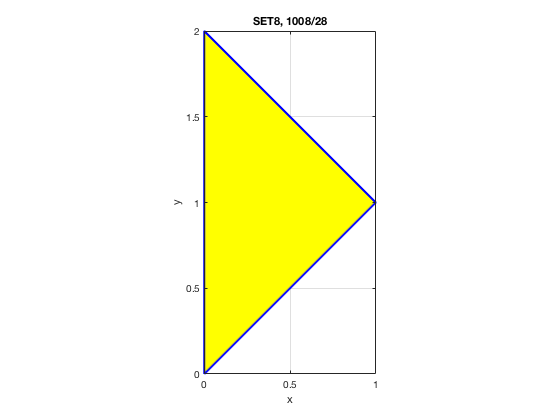V = int(int(x-0, y, x, 2-x), x, 0, 1)
V =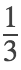V_appx = double(V) % in cm^3
V_appx = 0.3333

#### 5. [1009/36]

The volume of a solid bounded by three surfaces is computed.
syms x y z
f(x) = x^2 % y = x^2 is the parabolic cylinder
f(x) =% Here are two planes and their line of intersection r(x).
plane1 = z == 3*y, plane2 = z == 2+y, L = solve([plane1 plane2], [y z])
plane1 =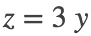plane2 =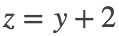L = struct with fields:
y: [1×1 sym] z: [1×1 sym]
r(x) = [x L.y L.z] % Project this line down onto xy-plane: y = 1, x free.
r(x) =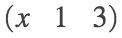x_bounds = solve(x^2 == 1, x) % y_bounds: x^2 <= y <= 1. Note that y is nonnegative here: 0 <= y <= 1.
x_bounds =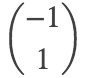% Note plane2 is above plane1: e.g., plug y = 1/2 into plane equations.
V = int(int(2+y - 3*y, y, x^2, 1), x, -1, 1) % volume of solid
V =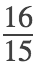V_appx = double(V) % in cm^3
V_appx = 1.0667
figure
fsurf(x, y, 2+y, [-1 1 -0.25 1.25], 'r', 'MeshDensity', 10); hold on % red plane z = 2+y above
fsurf(x, y, 3*y, [-1 1 -0.25 1.25], 'g', 'MeshDensity', 10) % green plane z = 3*y below
fsurf(x, x^2, z, [-1.25 1.25 -1 4], 'y', 'MeshDensity', 16) % yellow parabolic cylinder on side
xlabel('x'); ylabel('y'); zlabel('z')
title('SET8, 1009/36: 3D plot of surfaces')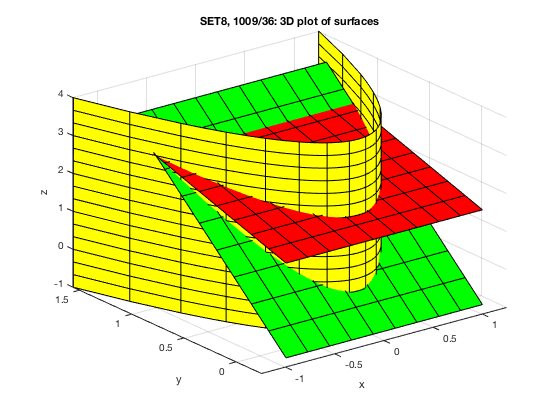%
figure
xs = linspace(-1,1); ys = xs.^2;
X = [xs xs(1)]; Y = [ys ys(1)];
fill(X,Y,'y'); grid on; hold on
plot(X,Y,'b', 'LineWidth', 2)
plot([-1 1], [0 0], 'k')
plot([0 0], [-0.5 1.5], 'k')
axis equal; axis([-1 1 -0.5 1.5])
xlabel('x'); ylabel('y')
xticks(-1:1); yticks(0:1)
title('SET8, 1009/36: 2D plot of integration region')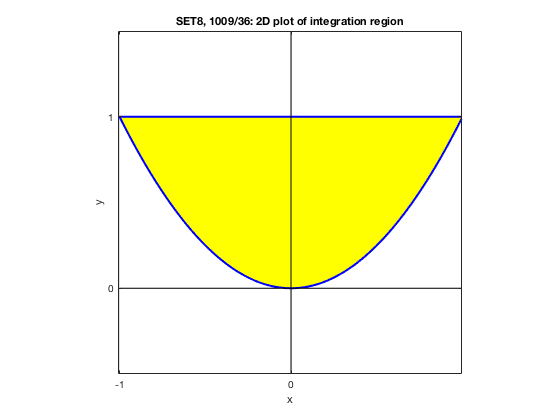#### 6. [1009/42]

The volume between two elliptic paraboloid and inside a circular cylinder is determined.
syms r x y z theta
LP = z == 2*x^2 + y^2, UP = z == 8 - x^2 - 2*y^2 % lower and upper paraboloids
LP =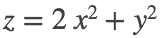UP =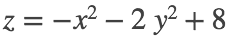f = rhs(LP), g = rhs(UP), s = sqrt(1-x^2), s83 = sqrt(8/3)
f =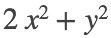g =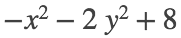s =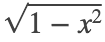s83 = 1.6330
CC = x^2 + y^2 == 1 % circular cylinder
CC =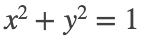V = int(int(g-f, y, -s, s), x, -1, 1)
V =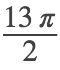V_appx = double(V) % in cm^3
V_appx = 20.4204
% Looking ahead to Section 15.3, we'll draw a nicer plot via polar coordinates.
ct = cos(theta), st = sin(theta), xp = r*ct, yp = r*st
ct =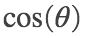st =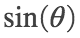xp =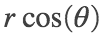yp =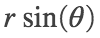fp = simplify(subs(f, [x y], [xp yp]))
fp =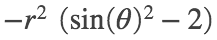gp = simplify(subs(g, [x y], [xp yp]))
gp =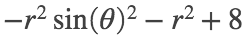%
figure
fsurf(xp, yp, gp, [0 s83 0 2*pi], 'r', 'MeshDensity', 20); hold on % red paraboloid z = 8 - x^2 - 2*y^2 above
fsurf(xp, yp, fp, [0 s83 0 2*pi], 'g', 'MeshDensity', 20) % green paraboloid z = 2*x^2 + y^2 below
fsurf(ct, st, z, [0 2*pi -1 8], 'y', 'MeshDensity', 20) % yellow circular cylinder x^2 + y^2 = 1 on side
xlabel('x'); ylabel('y'); zlabel('z')
xticks(-1:1); yticks(-1:1); zticks(0:4:8)
title('SET8, 1009/42: 3D plot of surfaces')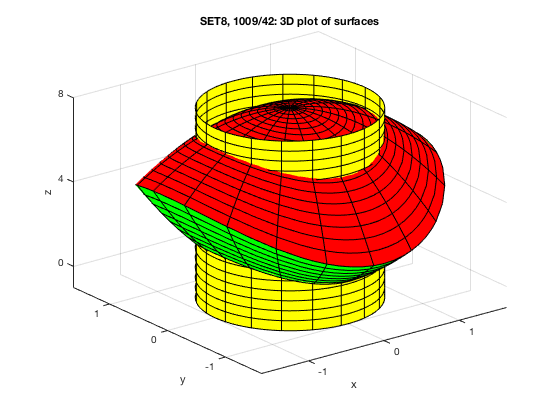#### 7. [1009/50]

Changing the order of integration, we have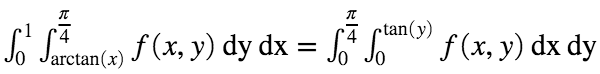. (See figure!)
figure
x = linspace(0,1); y = atan(x);
X = [x 0 0]; Y = [y pi/4 0];
fill(X,Y,'y'); grid on; hold on
plot(X,Y,'b', 'LineWidth', 2)
plot([-0.5 1.5], [0 0], 'k')
plot([0 0], [-0.5 1.5], 'k')
axis equal; axis([-0.5 1.5 -0.5 1.5])
xlabel('x'); ylabel('y')
xticks(0:1); yticks(0:1)
title('SET8, 1009/50: 2D plot of integration region')
text(0.5, 0.4, 'y = arctan(x) or x = tan(y)', 'FontSize', 12)
text(0.4, 0.9, 'y = \pi/4', 'FontSize', 12)
text(-0.25, 0.5, 'x = 0', 'FontSize', 12)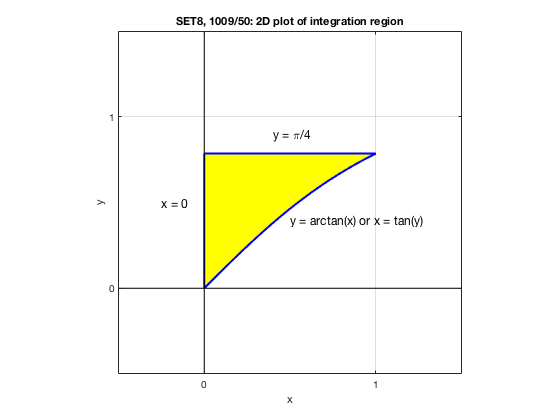#### 8. [1009/56]

Changing the order of integration, we have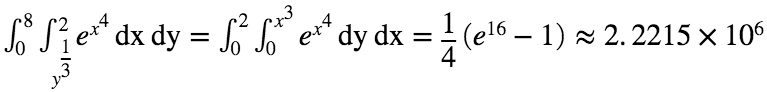. (See figure!)
syms x y
I = int(int(exp(x^4), y, 0, x^3), x, 0, 2)
I =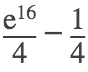I_appx = double(I)
I_appx = 2.2215e+06
%
figure
xs = linspace(0,2); ys = xs.^3;
X = [xs 2 0]; Y = [ys 0 0];
fill(X,Y,'y'); grid on; hold on
plot(X,Y,'b', 'LineWidth', 2)
plot([-2 4], [0 0], 'k')
plot([0 0], [-1 9], 'k')
axis equal; axis([-2 4 -1 9])
xlabel('x'); ylabel('y')
xticks(0:2:2); yticks(0:2:8)
title('SET8, 1009/56: 2D plot of integration region')
text(-1.25, 4.6, 'x = y^{1/3} or y = x^3', 'FontSize', 12)
text(0.6, -0.5, 'y = 0', 'FontSize', 12)
text(2.5, 3.8, 'x = 2', 'FontSize', 12)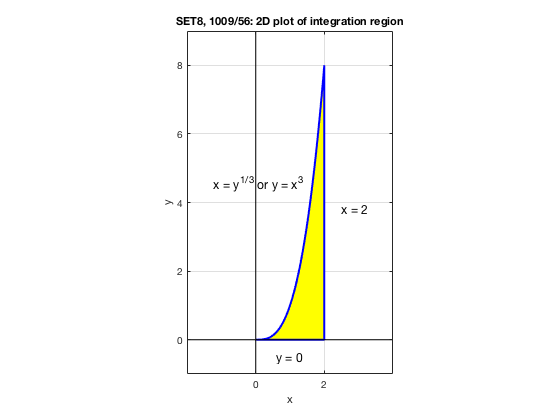#### 9. [1009/62]

RECALL from Section 15.1: The average value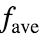of f over a region R is given by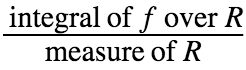.
• In Calculus 1, the region of integration was an interval. Hence the measure is the length of the interval.
• For a double integral in Calculus 3, the measure of a 2D region is its area.
• For a triple integral in Calculus 3, the measure of a 3D region is its volume.
syms x y
f(x,y) = x*sin(y)
f(x, y) =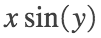I = int(int(f(x,y), y, 0, x^2), x, 0, 1)
I =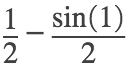A = int(int(1, y, 0, x^2), x, 0, 1) % Area is the double integral of 1 over the region.
A =A_alt = int(x^2 - 0, x, 0, 1) % Alternatively (in this instance), check it via Calc 1 techniques.
A_alt =f_ave = I/A
f_ave =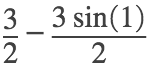f_ave_appx = double(f_ave)
f_ave_appx = 0.2378

#### 10. [1010/70]

Section 15.3 (Double Integrals in Polar Coordinates) is just around the corner. But why stop there? How about shifted polar coordinates?
syms r t x y z
plane = x + y + z == 1, paraboloid = z == 4 - x^2 - y^2
plane =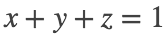paraboloid =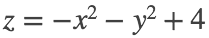eq1 = subs(paraboloid, z, 1-x-y) % The curve of intersection
eq1 =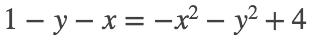eq2 = lhs(eq1) - rhs(eq1) == 0 % of the two surfaces is seen
eq2 =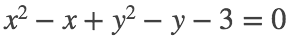eq3 = eq2 + 1/4 + 1/4 + 3 % to lie on a circular cylinder.
eq3 =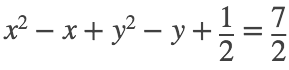c = (x-1/2)^2 + (y-1/2)^2 == 7/2 % an offset or shifted circle: Beauty, eh?
c =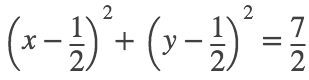% Now let's do the voodoo that you do SO WELL!
xsp = 1/2 + r*cos(t), ysp = 1/2 + r*sin(t) % shifted polar coordinates
xsp =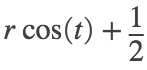ysp =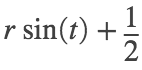f = 1 - xsp - ysp % Green plane is below (as you can see in the plot).
f =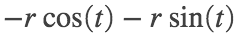g = 4 - xsp^2 - ysp^2 % Red paraboloid is above (the curved surface).
g =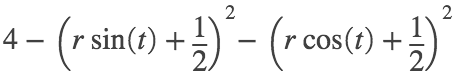V = int(int((g-f)*r, r, 0, sqrt(7/2)), theta, 0, 2*pi) % The factor of r is your polar JacFac.
V =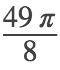% Yes, Studenten, that's a page off the Section 15.9 playbook, heuristically justified in 15.3.
V_appx = double(V) % in cm^3
V_appx = 19.2423
% This agrees with the double integral I did in rectangular coordinates, which is UGLY!
%
figure
fsurf(xsp, ysp, g, [0 sqrt(7/2) 0 2*pi], 'r', 'MeshDensity', 20); hold on % red paraboloid above
fsurf(xsp, ysp, f, [0 sqrt(7/2) 0 2*pi], 'g', 'MeshDensity', 10) % green plane below
xlabel('x'); ylabel('y'); zlabel('z')
axis equal
% xticks(-1:1); yticks(-1:1); zticks(0:4:8)
title('SET8, 1010/70: 3D plot of surfaces')
view(-40,44)